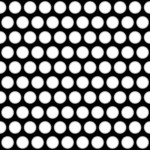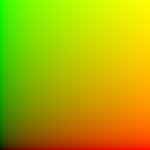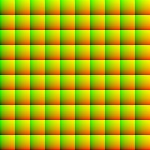Nuke Knowledge Base

Nuke stuff I need the interwebs to remember for me.

# Creating Procedural Textures via UV-Map with Expression-Node

To create cool procedural textures in nuke, there is a pretty simple way to do that, all you need is an input image, a STMap-node and an Expression-node.

The result we get looks something like this:As source image we use a simple white circle.To get the result above we have do to a little trick. We create a fake uv-image and apply it to the STMap, to get the desired result.

To create the UV-Map we use an Expression-node and some of the TCL-functions.

We are going to use floor() and (x%y).

What does floor() do?
It just rounds your numbers and cuts off the decimal digits.
So floor(1.45) = 1

What does (x%y) do?
This is called modulo or modulus. It divides x by y, and gives you the remainder.
So (3%2) = 1, (22%4) = 2, etc…

So how can we create a UV-map from this code? A UV-map looks like this:We have a red-gradient from right to left and a green-gradient from top to bottom.

We can also create the map above via expression. To do that we have to create an Expression-Node, apply it to a constant and write this:In this example, we used a 1000×1000 px Constant.

In the red channel, we write:

This gives us the remainder of the current x pixel divided by 1000. And to get a proper color representation we divide it by 1000.

In the green channel, we write:

This gives us the same result, just with the y-pixel-value.

Now we can start creating procedural textures. For example if we change the divider.
So we can use 100 instead of 1000.
This will give us the following uv-map:Now if we apply this to an STMap, we get our first result:This far, we could just use the Tile-node to get the same result.

So now that we can use the modulo-expession, it is time to use the floor()-expression, to create textures with offset.

To create an offset, we have to edit the red-channel. The green-channel can stay as it is. The red channel has to look like this:

So, what does this do?
We split the image in 10 different parts, by dividing it by 100. Then we use floor(), to get the absolute value. Then we want the modulo, to divide everything into black and white lines, to get two different areas.

So we use the if-statement to get two different cases.
The first one

is the normal step we used before. But the second one is different, we want these UVs to have an offset.

So we just add 50 to the x-value. The result we get should look like this:And if we apply this to the STMap, we get an result like this:With this you can create several procedural textures in nuke! Have fun!